Unit 4 part 3 Programming with C++ array Learn Online PDF Free Download

### ARRAYS :-

Array is a group of same data types variables which are referred by a common name.
An array is a series of elements of the same type placed in contiguous memory locations that can be individually referenced by adding an index to a unique identifier.

##### Need for Arrays :-

Suppose we have to store roll number & marks of 50 students in a program , then in all we need 100 variable each having a different name. Now remembering and managing these 100 variables is not easy task and it will make the program a complex and notunderstandable program.
But if we declare two array each having fifty elements one for roll numbers and another for marks. Now, we have only two names to remember. Elements of these array will be referred to as Arrayname[n], where n is the element number in in the array . Therefore arrays are very much useful in a case where quit many elements of the same data types need to be stored and processed. The element number s in parenthesis are called subscripts or index. The subscript, or index of an element designates its position in the array’s ordering.

###### Types of Array :-

Arrays are of two types:
1. One-dimensional Arrays
2. Multi-dimensional Arrays (But we have to discussing only Two-dimensional arrays in our syllabus

###### One –dimensional Arrays :-

The simplest form of an array is one - dimensional. The array itself is given a name and its elements are referred to by their subscripts. In C++ , an array must be defined before it can be used to store information. The general form of an array declaration is:Data_type Array_name[size];
For example the following statement declares an array marks of int data type , which have 50 elements marks to marks. int marks ;

##### Referencing Array Elements:-

We can reference array elements by using the array’s name& subscript (or index). The first element has a subscript of 0. The last element in an array of length n has a subscript of n-1. If we declare an array: int scores; then its 10 element will be referred as:
scores, scores, ……………………………….., scores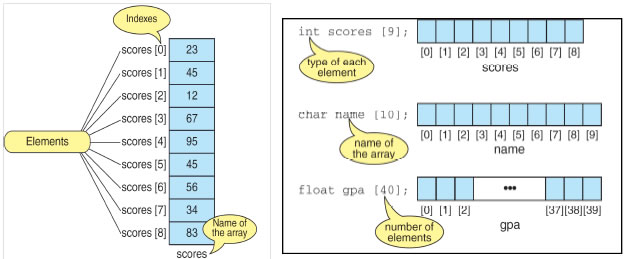##### Memory Representation of Single Dimensional Arrays:-

Elements of single dimensional array are stored in contiguous memory location in their index order. For example , an array p of type char with 6 elements declared as: char p; will be represented in memory as shown below: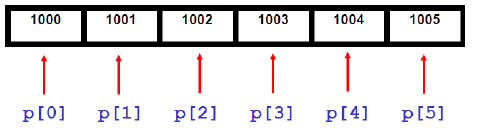If we declare an int array age with 5 elements as: int age;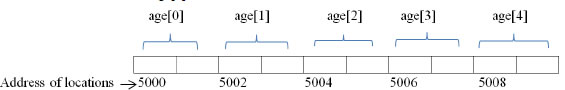Accepting Data in Array from User (Inputting Array elements) and Printing Array elements:- Since we can refer to individual array elements using numbered indexes, it is very common for programmers to use for (or any) loops when processing arrays.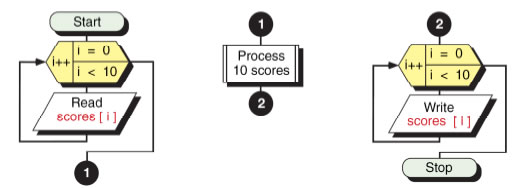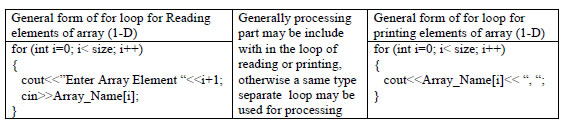Program : A C++ program to read an array of 10 elements and print the sum of all elements of array:
#include <iostream.h>
void main()
{
int a, sum=0;
for(int j=0; j<10; j++) //loop for reading
{
cout<< “Enter Element “<< j+1<<”:”;
cin>> a[j]
sum=sum+a[j]`;
}
cout<<”Sum : “<<sum;
}

Program: Here’s another example of an array this program , invites the user to enter a series of six values representing sales for each day of the week (excluding Sunday), and then calculates the average sales

#include <iostream.h>
void main()
{
const int SIZE = 6; //size of array
double sales[SIZE]; //array of 6 elements
double total = 0;
cout<< “Enter sales for 6 days\n”;
for(int j=0; j<SIZE; j++) //put figures in array
cin>> sales[j];
for(j=0; j<SIZE; j++) //read figures from array
total += sales[j]; //to find total
double average = total / SIZE; // find average
cout<< “Average = “ << average << endl;
}
Output:-
Enter sales for 6 days
352.64
867.70
781.32
867.35
746.21
189.45
Average = 634.11

###### Linear Search:-

In linear search, each element of the array is compared with the given item to be searched for , one by one either the desired element is found or the end of the array is reached.

#include<iostream.h>
void main( )
{
int size, i, n, c=0;
cout<<”Enter the size of array:”
cin>>size;
int a[size];
cout<<”Enter the elements of Array: “;
58
for(i-0; i<size; i++)
{
cin>>a[i];
}
cout<<” Enter the number to be search : “;
cin>>n ;
for (i=0; i<size; i++)
{ if (a[i]= =n)
{
c=1;
break;
}
}
if (c= =0)
cout<<” The number is not in the array.”;
else
cout<<” The Number is found and location of element is: “<<i+1:
}

###### Initializing arrays.

When we declare an array, we have the possibility to assign initial values to each one of its elements by enclosing the values in braces { }. Syntax for initialization of one-dimensional array is:

Data_type Array_name[sise] = { value list } ;
For example:
int num  = { 16, 2, 77, 40, 12071 };
This declaration would have created an array like this: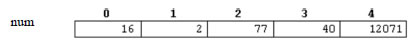The amount of values between braces { } must not be larger than the number of elements that we
declare for the array between square brackets [ ].
C++ allows us to skip the size of the array in an array initialization statement ( by leaving the
square brackets empty [ ] ). In this case, the compiler will assume a size for the array equal to the
number of values given between braces { }:
int num [ ] = { 16, 2, 77, 40, 12071 };
After this declaration, the size of array num will be 5, since we have provided 5 initialization
values.

Program : Program to find the maximum and minimum of an array :
#include<iostream.h>
void main( )
{
int arr[ ] = {10, 6 , -9 , 17 , 34 , 20 , 34 ,-2 ,92 ,22 };
int max = min = arr;
for(int i = 0 ; i<10 ; i++)
{
if(arr[i] < min)
min= arr[i];
if(arr[i] > max)
max = arr[i];
}
cout<<”The minimum of all is : “ << min<<”and the maximum is : “ <<max;
}

String as an array:- C++ does not have a string data type rather it implements string as single dimensional character array. A string is defined as a character array terminated by a null character ‘\0’. Hence to declare an array strg that holds a 10 character string, you would write : char strg; Program : Program to count total number of vowels present in a string :

#include<iostream.h>
#include<stdio.h>
void main( )
{
char string; int count = 0;
cout<<”Input a string”;
gets(string); // library function in stdio.h to input a string
for(int i = 0 ; string[i] != '\0' ; i++)
{
if( string[i] = = 'a' || string[i] = = 'e' || string[i] = = 'o' || string[i] = = 'u' || string[i] = = 'i' ||
string[i] = = 'A' || string[i] = = 'E' || string[i] = = 'O' || string[i] = = 'U' || string[i] == 'I' )
{
count++;
}
}
}

Two dimensional array : A two dimensional array is a continuous memory location holding similar type of data arranged in row and column format (like a matrix structure).Declaration of 2-D array:- The general syntax used for 2-Darray declaration is:
Data_type Array_name [R][C] ;
Where R represent number of rows and C represent number of columns in array.
For example,
int marks ;
and, for example, the way to reference the second element vertically and fourth horizontally in an expression would be: marks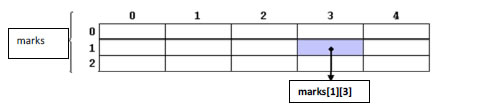Initialization of 2-D Array:- Two dimensional arrays are also initialize in the same way as single dimensional array . e. g.,
int cube = {1,1, 2,8,3,27,4,64,4,125 };
will initialize cube=1, cube=1, cube=2, cube=8 , cube=3 and so on.

2-D array can also be initialized in unsized manner. However the first index can be skiped , the second index must be given. e.g., int cube[ ] = {1,1, 2,8,3,27,4,64,4,125 }; is also valid

Accepting Data in 2-D Array from User (Inputting 2-D Array elements) and Printing 2-D Array elements:- Since We must reference both, a row number and a column number to input or output values in a two-dimensional array. So, we use a nested loop (generally nested for loop) , when processing 2-D arrays.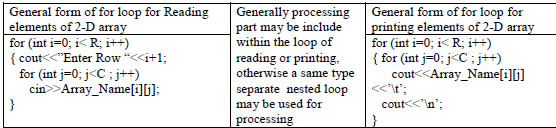Where R represent number of rows and C represent number of columns in array.
Program : Program to subtract two 3x3 matrices and then print the resultant matrix.

#include <iostream.h>
#include<conio.h>
main()
{
int m, n, c, d, first, second, sub;
cout<<"Enter the elements of first matrix\n";
for ( i = 0 ; j < 3 ; i++ )
for ( j = 0 ; j <3 ; j++ )
cin>>first[i][j];
cout<<"Enter the elements of second matrix\n";
for ( i = 0 ; j < 3 ; i++ )
for ( j = 0 ; j <3 ; j++ )
cin>>second[i][j];
cout<<"Subtraction of entered matrices:-\n";
for ( i = 0 ; j < 3 ; i++ )
{
for ( j = 0 ; j <3 ; j++ )
{
sub[i][j] = first[i][j] - second[i][j];
cout<<sub[i][j]<<’\t’;
}
cout<<’\n’ ;
}
getch();
}

###### Array of Strings:-

An array of string is a two-dimensional character array. The size of first index determines the number of strings and size of second index determines maximum length of each string. The following statement declares an array of 10 strings , each of which can hold maximum 50 valid characters. char string ; Notice that the second index has been given 51, because 1 extra size is given for store null character ‘\0’.

###### User Defined Data Types:-

The C++ language allows you to create and use data types other than the fundamental data types. These types are called user-defined data types. Structures:- In C++, a structure is a collection of variables that are referenced by a single name. The variable can be of different data types. They provide a convenient means of keeping related information together.

###### Defining Structure :-

A Structure is defined by using the Keyword struct. The General Syntax for defining a Structure is :

Syntax :
struct< Name of Structure >
{
<datatype>< data-member 1>;
<datatype>< data-member 2>;
<datatype>< data-member 3>;

<datatype>< data-member n>;
} ;
Example :
A Proper example following the previous syntax could be :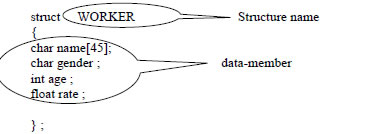### NCERT Books Free Pdf Download for Class 5, 6, 7, 8, 9, 10 , 11, 12 Hindi and English Medium

 Mathematics Biology Psychology Chemistry English Economics Sociology Hindi Business Studies Geography Science Political Science Statistics Physics Accountancy